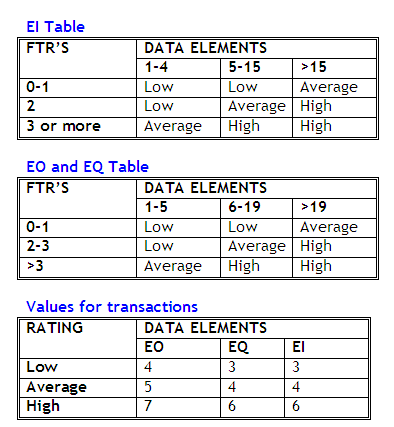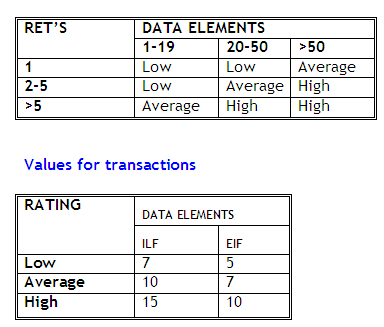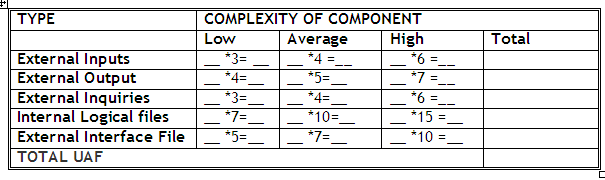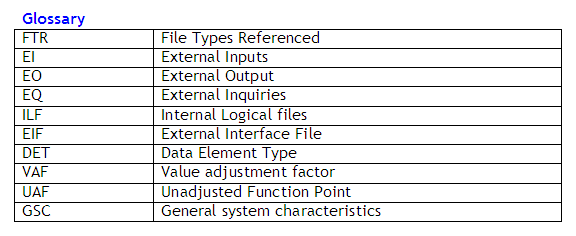Post

# Fundamentals of Function Point Analysis Part II

Each of the following tables assists in the ranking process. For example, an EI that references or updates 2 File Types Referenced (FTR's) and has 7 data elements would be assigned a ranking of average and associated rating of 4. Where FTR's are the combined number of Internal Logical Files (ILF's) referenced or updated and External Interface Files referencedFor both ILF's and EIF's the number of record element types and the number of data elements types are used to determine a ranking of low, average or high. A Record Element Type is a user recognizable subgroup of data elements within an ILF or EIF. A Data Element Type (DET) is a unique user recognizable, non recursive field on an ILF or EIF.Each count is multiplied by the numerical rating shown to determine the rated value. The rated values on each row are summed across the table, giving a total value for each type of component. These totals are then summed across the table, giving a total value for each type of component. These totals are then summoned down to arrive at the Total Number of Unadjusted Function Points (UAF).The value adjustment factor (VAF) is based on 14 general system characteristics (GSC's).
The degrees of influence range on a scale of zero to five, from no influence to strong influence.

1. Data communication
2. Distributed functions
3. Performance objectives
4. Heavily used configuration
5. Transaction rate
6. On-line data entry
7. End-user efficiency
8. On-line update
9. Complex processing
10. Reusability
11. Installation ease
12. Operational ease
13. Multiple sites
14. Facilitate change

VAF = 0.65 + ( sum of 14 characteristics ) / 100

The final Function Point Count is obtained by multiplying the VAF times the Unadjusted Function Point (UAF).

FP = UAF * VAFRecommended Free Ebook
Similar Articles